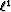Next: 2-D data example: attenuation Up: Principles of norm and Previous: Shaping filters and the

### Results on a simple 1-D example

For the simple 1-D example under consideration, the filter coefficients are now estimated with thenorm approximated with the Huber function. In Figure, I display the result of the adaptive subtraction when thenorm is utilized to estimate a single shaping filter (equation () with a small).1Dl1
Figure 5
(a) The signal estimated with thenorm. (b) The noise estimated with thenorm.The estimated signal in Figurea resembles the true signal very well, and so does the estimated noise. It is easy to check that the energy (in asense) in Figurea (e1=2) is less than the energy (in asense) in Figurea (e1=3.2). Figureshows the shaping filter associated with thenorm. This filter is a spike at lag=0. This simple 1-D example demonstrates that thenorm should be utilized whenever significant amplitude differences exist between multiples and primaries. In the next section, a synthetic example where internal multiples are attenuated illustrates the properties of thesubtraction.Next: 2-D data example: attenuation Up: Principles of norm and Previous: Shaping filters and the
Stanford Exploration Project
5/5/2005